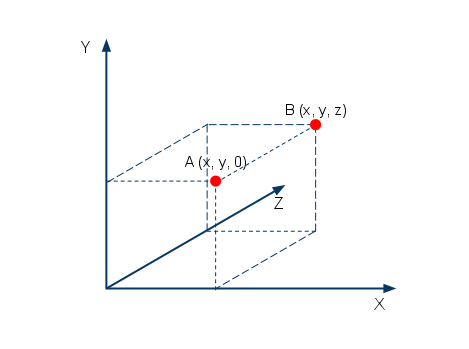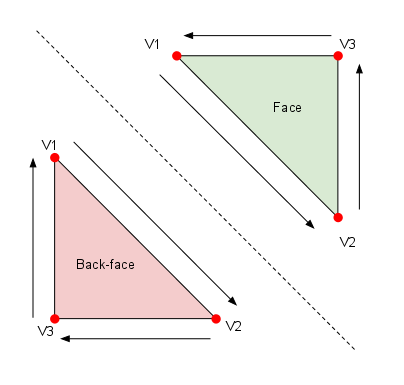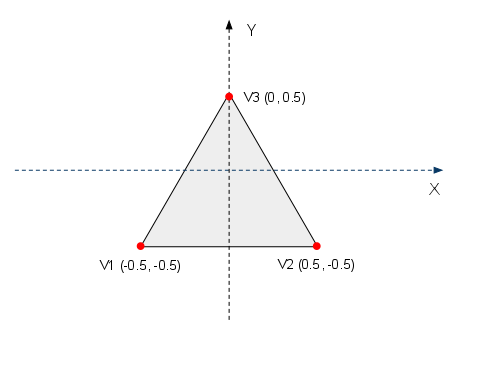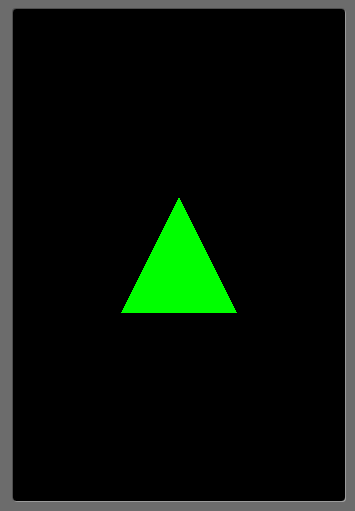# Displaying Graphical Elements (Primitives) with OpenGL ES

This is part 2 of the android OpenGL ES series. In the previous article we looked at how to set up the android project to use the provided OpenGL view with our renderer. You can use the project from that article as a template for this.

Before we start displaying things, we must know a few basic concepts of 3D programming and also familiarise ourselves with the terminology. I’s basic geometry really.

3D graphics happens in the Cartesian Coordinate System.

That means that the coordinate system used has three dimensions. `X`, `Y` and `Z`.

Traditionally `X` goes from left to right, `Y` from bottom to top, and `Z` from me into the screen so to speak.

While we deal with objects to display (a robot for example or a car) OpenGL deals with components of these objects. Each object is created from `primitives` which in the case of OpenGL is a `triangle`. Every triangle has a `face` and a `backface`.

A triangle is defined by 3 points in space. A point is called a `vertex` (`vertices` plural).

The following diagram shows 2 vertices. `A` and `B`.Vertices

I drew this diagram to show how we will differentiate between 2D and 3D. A vertex is defined by its X, Y and Z coordinates. If we use 0 for the Z component all the time, we have 2D. You can see vertex A is part of the plane defined by X and Y. Vertex B is farther in on the Z coordinate. If you think of Z as a line being perpendicular to the screen, we wouldn’t even see B.

A `triangle` is called a `primitive`. A primitive is the simplest type OpenGL understands and is able to graphically represent.

It is very simple. 3 vertices define a triangle. There are other primitives as well, like quads but we’ll stick to the basics. Every shape can be broken down into triangles.

We mentioned the `face` of the triangle before.

Why is it important? In 3D you will have objects with parts facing towards you, the player and parts facing away from you. In order to make the drawing efficient, OpenGL will not draw the triangles facing away from you as it is not necessary because they will be hidden by the triangles facing towards you anyway. This is called backface culling.

How does OpenGL determine this? This is determined by the order of the vertices when drawing the triangle. If the order is counter clockwise then it is a face (green triangle). Clockwise order of the vertices means it is a backface (the red triangle). This is the default setting but it can be changed of course. The following diagram illustrates just that.Backface Culling

The red triangle won’t be drawn.

## Creating and Drawing a Triangle

With all the theory understood, let’s create a triangle and draw it.

A triangle is defined by 3 vertices. The coordinates of the vertices is not measured in pixels. We will use `float` to represent the values and they will be relative to each other.

If a side’s length is `1.0f` and an other side’s length is `0.5f`, then it means that the second side is half the length of the first side’s length. How big it will be displayed depends on how the viewport is set up. Imagine the viewport as a camera. When we use 2D then it means that the camera is orthogonal to the screen. If the camera is very close, the triangle will appear big, if it’s far, then the triangle will be small.

Let’s create the `Triangle` class.

``````
package net.obviam.opengl;

import java.nio.ByteBuffer;

import java.nio.ByteOrder;

import java.nio.FloatBuffer;

import javax.microedition.khronos.opengles.GL10;

public class Triangle {

private FloatBuffer vertexBuffer;   // buffer holding the vertices

private float vertices[] = {

-0.5f, -0.5f,  0.0f,        // V1 - first vertex (x,y,z)

0.5f, -0.5f,  0.0f,        // V2 - second vertex

0.0f,  0.5f,  0.0f         // V3 - third vertex

};

public Triangle() {

// a float has 4 bytes so we allocate for each coordinate 4 bytes

ByteBuffer vertexByteBuffer = ByteBuffer.allocateDirect(vertices.length * 4);

vertexByteBuffer.order(ByteOrder.nativeOrder());

// allocates the memory from the byte buffer

vertexBuffer = vertexByteBuffer.asFloatBuffer();

// fill the vertexBuffer with the vertices

vertexBuffer.put(vertices);

// set the cursor position to the beginning of the buffer

vertexBuffer.position(0);

}

}

``````

Line 11 defines a `FloatBuffer` that will hold the vertices for our triangle. We need to use the `java.nio` package as it is very intensive input output.

The `vertices[]` array holds the actual coordinates for the vertices.

The triangle we will draw is represented in the following diagram. We calculate everything from the origin.Triange

In the constructor we initialise the triangle from this `vertices[]` array.

What we do is, we fill the `vertexBuffer` with the coordinates and set the cursor’s position to the beginning of the buffer. We will be using this buffer in the OpenGL call to display triangle strips. We currently have just one.

Let’s take a look at the renderer. The `GlRenderer`

``````
package net.obviam.opengl;

import javax.microedition.khronos.egl.EGLConfig;

import javax.microedition.khronos.opengles.GL10;

import android.opengl.GLU;

import android.opengl.GLSurfaceView.Renderer;

public class GlRenderer implements Renderer {

private Triangle    triangle;   // the triangle to be drawn

/** Constructor */

public GlRenderer() {

this.triangle = new Triangle();

}

@Override

public void onDrawFrame(GL10 gl) {

// clear Screen and Depth Buffer

gl.glClear(GL10.GL_COLOR_BUFFER_BIT | GL10.GL_DEPTH_BUFFER_BIT);

// Reset the Modelview Matrix

gl.glLoadIdentity();

// Drawing

gl.glTranslatef(0.0f, 0.0f, -5.0f);     // move 5 units INTO the screen

// is the same as moving the camera 5 units away

triangle.draw(gl);                      // Draw the triangle

}

@Override

public void onSurfaceChanged(GL10 gl, int width, int height) {

if(height == 0) {                       //Prevent A Divide By Zero By

height = 1;                         //Making Height Equal One

}

gl.glViewport(0, 0, width, height);     //Reset The Current Viewport

gl.glMatrixMode(GL10.GL_PROJECTION);    //Select The Projection Matrix

gl.glLoadIdentity();                    //Reset The Projection Matrix

//Calculate The Aspect Ratio Of The Window

GLU.gluPerspective(gl, 45.0f, (float)width / (float)height, 0.1f, 100.0f);

gl.glMatrixMode(GL10.GL_MODELVIEW);     //Select The Modelview Matrix

gl.glLoadIdentity();                    //Reset The Modelview Matrix

}

@Override

public void onSurfaceCreated(GL10 gl, EGLConfig config) {

}

}

``````

We create the triangle in the constructor.

The `onDrawFrame(GL10 gl)` is of the most interest for us.

OpenGL works with state variables. Every method we call on the OpenGL context changes its internal state.

Following the `onDrawFrame` method we see that every time a frame is drawn, the buffers get cleared, the ModelView matrix is reloaded (don’t worry if you don’t understand this at the moment), the camera is moved away 5 units (we’re dealing with units here, not pixels) and the triangle’s `draw()` method is called.

The `onSurfaceChanged` on the other hand, transitions the OpenGL context between a few states. First it sets the viewport to the current width and height of the surface (so it works with the `GL_PROJECTION` state), then it transitions the state to the `GL_MODELVIEW` so we can work with our models – the triangle in our case. It will make sense later on, don’t worry.

Let’s check out the `draw` method for the triangle:

``````
public void draw(GL10 gl) {

gl.glEnableClientState(GL10.GL_VERTEX_ARRAY);

// set the colour for the triangle

gl.glColor4f(0.0f, 1.0f, 0.0f, 0.5f);

// Point to our vertex buffer

gl.glVertexPointer(3, GL10.GL_FLOAT, 0, vertexBuffer);

// Draw the vertices as triangle strip

gl.glDrawArrays(GL10.GL_TRIANGLE_STRIP, 0, vertices.length / 3);

//Disable the client state before leaving

gl.glDisableClientState(GL10.GL_VERTEX_ARRAY);

}

``````

Because we store the triangle’s vertices’ coordinates in a `FloatBuffer` we need to enable OpenGL to read from it and understand that is a triangle there. Line 02 does just that.

Line 05 sets the colour for the entity (triangle in our case) that will be drawn. Note that the values of the rgb are floats and are between 0.0 and 1.0.

`gl.glVertexPointer(3, GL10.GL_FLOAT, 0, vertexBuffer)` will tell OpenGL to use the `vertexBuffer` to extract the vertices from.

The first parameter (value = 3) represents the number of vertices in the buffer. The second lets OpenGL know what type the data the buffer holds.

The third parameter is the offset in the array used for the vertices. Because we don’t store extra data, our vertices follow each other and there is no offset.

Finally the last parameter is our buffer containing the vertices.

`gl.glDrawArrays(GL10.GL_TRIANGLE_STRIP, 0, vertices.length / 3)` tells OpenGL to draw triangle strips found in the buffer provided earlier, starting with the first element. It also lets it know how many vertices there are.

That is it. Run the project and you should be able to see your first accelerated triangle. Just like this:Triangle

Download the source here (TBD):